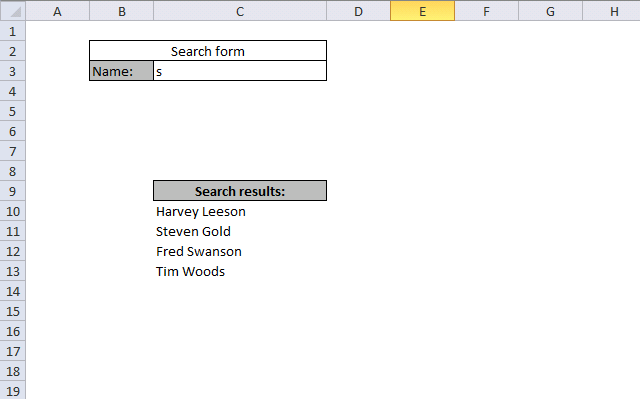Author: Oscar Cronquist Article last updated on December 02, 2018

Question: How do i create a flexible search formula to search a list?

Answer: The following formula let´s you search for a text string in a data set. Cells containing the text string are returned in cell range C10:C20.

Example, Cell C3 contains the text string "s". Names that contain text string s are instantly shown in cell range C10:C20.

Sheet: SearchSheet "Names"Array formula:

=IFERROR(INDEX(Names!\$A\$2:\$A\$9, SMALL(IF(ISERROR(SEARCH(Search!\$C\$3, Names!\$A\$2:\$A\$9)), "", MATCH(ROW(Names!\$A\$2:\$A\$9), ROW(Names!\$A\$2:\$A\$9))), ROW(A1))), "")

How to enter an array formula

1. Select cell C10
2. Click in formula bar
3. Type above formula
4. Press and hold CTRL + SHIFT simultaneously
5. Press Enter
6. Release all keys

If you did it right, the formula is now surrounded by curly brackets:

{=IFERROR(INDEX(Names!\$A\$2:\$A\$9, SMALL(IF(ISERROR(SEARCH(Search!\$C\$3, Names!\$A\$2:\$A\$9)), "", MATCH(ROW(Names!\$A\$2:\$A\$9), ROW(Names!\$A\$2:\$A\$9))), ROW(A1))), "")}

### Explaining array formula in cell C10

Step 1 - Search for a specific text string in a range

SEARCH(Search!\$C\$3, Names!\$A\$2:\$A\$9))

becomes

SEARCH("s", {"Name"; "Richard Dent"; "Harvey Leeson"; "Mick Rooney"; "Steven Gold"; "Peter McDonald"; "Fred Swanson"; "Jack Door"; "Tim Woods"; 0})

and returns

{#VALUE!; 11; #VALUE!; 1; #VALUE!; 6; #VALUE!; 9}

Step 2 - Check if an error is returned

ISERROR(SEARCH(Search!\$C\$3, Names!\$A\$2:\$A\$9))

becomes

ISERROR({#VALUE!; 11; #VALUE!; 1; #VALUE!; 6; #VALUE!; 9})

and returns

{TRUE; FALSE; TRUE; FALSE; TRUE; FALSE; TRUE; FALSE}

Step 3 - Create row numbers for those values that are FALSE

IF(ISERROR(SEARCH(Search!\$C\$3, Names!\$A\$2:\$A\$9)), "", MATCH(ROW(Names!\$A\$2:\$A\$9), ROW(Names!\$A\$2:\$A\$9)))

becomes

IF({TRUE; FALSE; TRUE; FALSE; TRUE; FALSE; TRUE; FALSE}, "", {1;2;3;4;5;6;7;8})

and returns

{"";2;"";4;"";6;"";8}

Step 4 - Return the k-th smallest row number

SMALL(IF(ISERROR(SEARCH(Search!\$C\$3, Names!\$A\$2:\$A\$9)), "", MATCH(ROW(Names!\$A\$2:\$A\$9), ROW(Names!\$A\$2:\$A\$9))), ROW(A1))

becomes

SMALL({"";2;"";4;"";6;"";8}, ROW(A1))

becomes

SMALL({"";2;"";4;"";6;"";8}, 1)

and returns

2

Step 5 - Return name

INDEX(Names!\$A\$2:\$A\$9, SMALL(IF(ISERROR(SEARCH(Search!\$C\$3, Names!\$A\$2:\$A\$9)), "", MATCH(ROW(Names!\$A\$2:\$A\$9), ROW(Names!\$A\$2:\$A\$9))), ROW(A1)))

becomes

INDEX(Names!\$A\$2:\$A\$9, 2)

becomes

INDEX({"Richard Dent"; "Harvey Leeson"; "Mick Rooney"; "Steven Gold"; "Peter McDonald"; "Fred Swanson"; "Jack Door"; "Tim Woods"}, 2)

and returns

Harvey Leeson in cell C10.